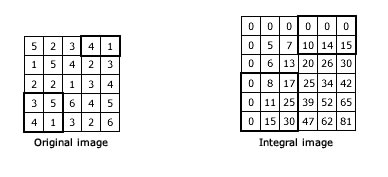## Introduction

An integral image lets you calculate summations over image subregions. Rapidly.These summations are useful in many applications, like calculating HAAR wavelets. These are used in face recognition and other similar algorithms.

## How it works

Suppose an image is w pixels wide and h pixels high. Then the integral of this will be w+1 pixels wide and h+1 pixels high. The first row and column of the integral image are all zeros.

All other pixels have a value equal to the sum of all pixels before it.See the integral in the above image? Every pixel is the summation of the pixels before it (above and to the left).

Now, to calculate the summation of the pixels in the black box, you take the corresponding box in the integral. You sum as follows: (Bottom right + top left - top right - bottom left).

So for the 3,5,4,1 box, the calculations would go like this: (30+0-17-0 = 13). For the 4,1 box, it would be (0+15-10-0 = 5).

This way, you can calculate summations in rectangular regions rapidly.

## More than just summations!

With the basic idea in mind, you can extend it to more types of summations. You can calculate the sum of squares. You can rotate the image by 45 degrees and then do the summations. Then, you can calculate the totals in any arbitrary rectangular region that is upright or tilted at 45 degrees.

You can calculate summations on irregular areas too (only those with 90 degree corners though). Not just that, you can do super fast blurs, approximate gradients and compute means and standard deviations very fast.

## Calculating Integral Images in OpenCV

OpenCV comes with a predefined function to calculate an integral image.

```void cvIntegral(const CvArr* image,
CvArr* sum,
CvArr* sqsum=NULL,
CvArr* tilted_sum=NULL);
```

The parameters are, as always, self explanatory:

• `image`: the source image
• `sum`: the sum summation integral image
• `sqsum`: the square sum integral image
• `tiled_sum`: image is rotated by 45 degrees and then its integral is calculated

## Summary

Calculating integral images is trivial. But they let you do more complex stuff (like blurring, HAAR wavelets, etc) super fast. And `cvIntegral` in OpenCV calculates integral images for you.

#### Related posts#### 7 unique neural network architectures#### An introduction to contours

Utkarsh Sinha created AI Shack in 2010 and has since been working on computer vision and related fields. He is currently at Microsoft working on computer vision.